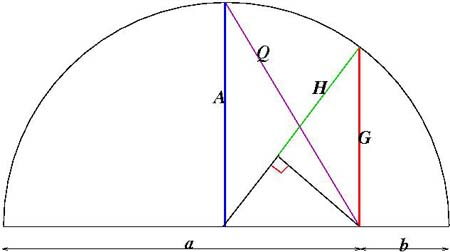#### You may also like### Shades of Fermat's Last Theorem

The familiar Pythagorean 3-4-5 triple gives one solution to (x-1)^n + x^n = (x+1)^n so what about other solutions for x an integer and n= 2, 3, 4 or 5?### Exhaustion

Find the positive integer solutions of the equation (1+1/a)(1+1/b)(1+1/c) = 2### Code to Zero

Find all 3 digit numbers such that by adding the first digit, the square of the second and the cube of the third you get the original number, for example 1 + 3^2 + 5^3 = 135.

# Classical Means

##### Age 16 to 18Challenge Level

For any two numbers $a$ and $b$ three classical Pythagorean means are defined, the arithmetic mean $A$, the geometric mean $G$ and the harmonic mean $H$ such that:
\eqalign{ A &= \frac{1}{2}(a+b) \cr G &= \sqrt {ab}\cr H &= \frac{2}{\frac{1}{a}+\frac{1}{b}}.}
(i) Prove that $H=\frac{G^2}{A}$.(ii) In this diagram the semicircle has diameter $a+b$. Prove that the lengths $A$, $G$ and $H$ (shown in blue, red and green) are equal to the three means and deduce from the diagram the inequality $$A> G> H.$$

(iii) Prove from the diagram that the length $Q$ is equal to the quadratic mean (or root mean square) such that $$Q=\sqrt{\frac {a^2+b^2}{2}}.$$